Here’s How to Calculate Sharpe Ratio in Excel with Formula

In this article, I’m going to explain how to calculate one of the most famous ratios, among financial analysis, to measure risk in Microsoft Excel: the Sharpe Ratio.

According to Investopedia:

The Sharpe ratio tells us whether a portfolio's returns are due to smart investment decisions or a result of excess risk. Although one portfolio or fund can reap higher returns than its peers, it is only a good investment if those higher returns do not come with too much additional risk. The greater a portfolio's Sharpe ratio, the better its risk-adjusted performance has been. A negative Sharpe ratio indicates that a risk-less asset would perform better than the security being analyzed.”

The formula is the following: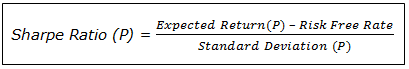Where  Expected Return (P) is the expected return of the portfolio and “Standard Deviation (P)” is the standard deviation of the portfolio.

Here’s How to Calculate Sharpe Ratio in Excel with Formula

Calculation of Sharpe Ratio

Now let’s get hands-on work and calculate the Sharpe Ratio for a two - stocks portfolio in MS Excel.

For the analysis, we will suppose that our portfolio is composed 50% by Apple Inc. (AAPL) and 50% by Microsoft Corp. (MSFT).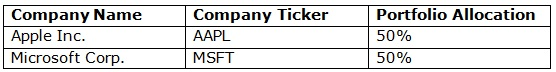Step 1:

First of all, we need to get the stocks’ daily prices in order to calculate the daily returns that we are going to use to calculate the variables that compose the Sharpe Ratio.

We can get the historical prices from many sources but the most famous ones are Yahoo! Finance or Google Finance: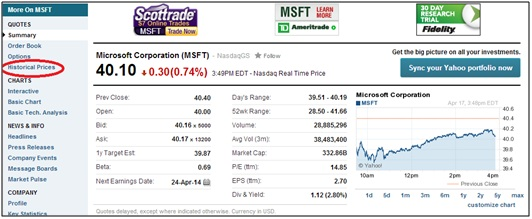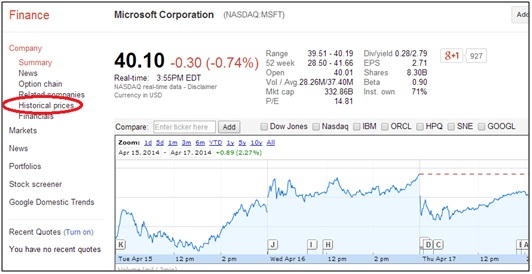Step 2:

Input the daily prices into an Excel worksheet and calculate daily returns for the period being analyzed (I will use a 3-year time horizon).

A daily return can be calculated with the formula below:

Daily Return = (Price 1 – Price 0) / Price 0

Daily Return = (Price 1 / Price 0) -1

Where Price 1 is the following day close price and Price 0 is the previous day close price.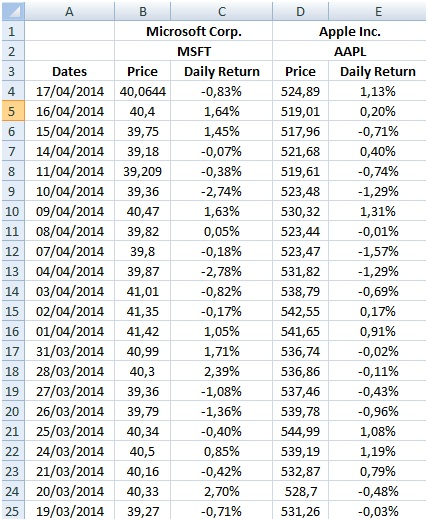Step 3:

Once we have all the daily returns we are able to calculate the expected return for each of the stocks. To do this, we have to calculate the average of the daily returns of the period being analyzed and then annualize it.

We will use Excel’s average formula: =AVERAGE (Range of Returns1) and then we annualize it by multiplying by 252 (tradable days).

Then, we have to do the same calculations for the second stock:

=AVERAGE (Range of Returns2) and then we annualize it by multiplying by 252.

Step 4:

Following the Sharpe Ratio formula, other variable we need to calculate is the Standard Deviation of the portfolio.

Let’s remember the Variance formula for a two-stocks portfolio:

VAR (P) = Weight1^2 * STDEV1^2 + Weight2^2 * STDEV^2 +2 * Weight1 * Weight2 * STDV1 * STDV2 *CORR(1,2)

Where COV(1,2) = STDEV1 *STDEV2 * CORR1,2

So, VAR (P) = Weight1^2 * STDEV1^2 + Weight2^2 * STDEV^2 + 2 * Weight1 * Weight2 * COV1,2

Then, the standard deviation of the portfolio is the squared root of the Variance.

STDEV (P) = (Weight1^2 * STDEV1^2 + Weight2^2 * STDEV^2 + 2 * Weight1 * Weight2 * COV1,2)^(1/2)

One of the inputs we can easily calculate in Excel is the Standard Deviation of the returns of each stock:

For the first stock = STDEV1 (Range of Returns1)

For the second stock = STDEV2 (Range of Returns2).

Then, we need to calculate the Covariance of the two stocks based on the returns.

Note: According to Investopedia, the covariance is a measure of the degree to which returns on two risky assets move in tandem. A positive covariance means that asset returns move together. A negative covariance means returns move inversely.

To do this we use the covariance function provided by Excel:  =COVAR (Range of Returns 1; Range of Returns2)

Once we have calculated the Standard Deviation and the Covariance of the stocks, we can put all the variables together and get the Standard Deviation of the Portfolio.

Suggested: Read more about excel for finance

The standard deviation calculated is in a daily basis and we have to get it annualized so we have to multiply the result by the squared root of 252 (average number of tradable days per year).

Step 5:

We are one step to finish the calculation! We just need to get the Risk Free Rate and put all the variables together. The United States Treasury Bonds are known as risk free because they always pay. For this analysis, a 5-year bond will be considered with an annual rate of 1.72%.

The numbers for the example are the following:

Annualized Expected Return MSFT= 0.07%*252 = 18.46%

Annualized Expected Return AAPL= 0.08%*252 = 19.29%

Portfolio’s Annualized Expeted Return= 0.5*18.46% + 0.5*19.29% = 18.87%

Std Dev MSFT= 1.49%

Std Dev AAPL= 1.77%

Covariance (MSFT, AAPL)= 0,0001

Portfolio’s Std Dev (Daily) = (0.5^2*1.49%^2+0.5^2*1.77%^2+2*0,5*0,5*0,0001)^(1/2) = 0.02%

Portfolio’s Std Dev (Annualized) = (0.02%*252)^(1/2)= 20.71%

Risk Free Rate= 1.72%

Putting all the variables together into the Sharpe Ratio formula we get the following: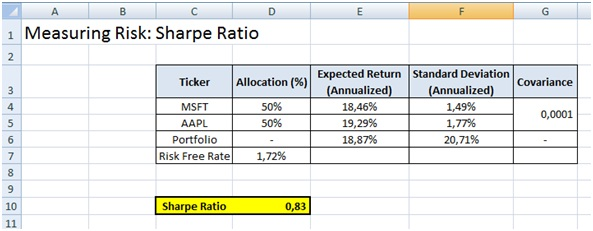Sharpe Ratio (P) = (18.87% - 1.72%) / 20.71% = 0.83

About the Author: Fernando Ventureira is a Financial Analyst at iBillionaire Inc.

Next Classroom Batches in India
- Classroom Course with Placement Assistance: Our Full-Time Financial Modeling Investment Banking Course (6 Weeks) starts on 11th November, 2019 and 2.5 Months Weekend Workshop starts on 27th September in New Delhi, India and on 14th December, 2019 in Gurgaon, India.

- Online Course with Placement Assistance: Join our Online Financial Modeling Investment Banking course with Placement Assistance from anywhere in the world.

- CFA Level - I: 16th November, 2019

- Big Data: 14th December, 2019

- Financial Modeling Investment Banking Weekend in Gurgaon: 14th December, 2019

- See Testimonials.

Only a few seats remain. Interested candidates can contact me.